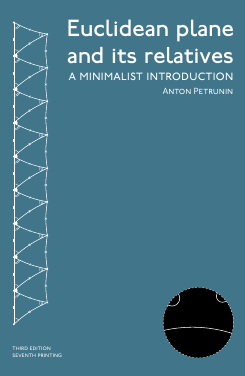# Euclidean plane and its relativesThe textbook is designed for a semester-long course in Foundations of geometry and meant to be rigorous, conservative, elementary and minimalist. It is based on lectures for course MATH 427 given at the Penn State University.

You can order a printed copy at amazon. Its exact copy can be downloaded at arXiv; printings of this (third) edition start with v16. If you use the printed version in class, make sure everyone gets the latest printing directly from amazon (some number-labels of exercises and theorems might be shifted in the older printings).

In addition to the traditional exercises in the book, one can use visual assignments.

Introduction
1. Preliminaries

Euclidean geometry
2. The Axioms
3. Half-planes
4. Congruent triangles
5. Perpendicular lines
6. Similar triangles
7. Parallel lines
8. Triangle geometry

Inversive geometry
9. Inscribed angles
10. Inversion

Non-Euclidean geometry
11. Neutral plane
12. Hyperbolic plane
13. Geometry of h-plane

14. Affine geometry
15. Projective geometry
16. Spherical geometry
17. Projective model
18. Complex coordinates
19. Geometric constructions
20. Area

Write me a short message if you are teaching a course using this book.

Write me if you see a mistake.